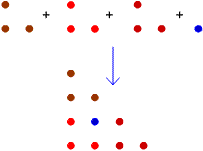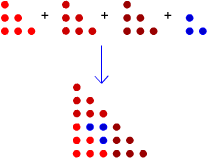#### You may also like### Diophantine N-tuples

Can you explain why a sequence of operations always gives you perfect squares?### DOTS Division

Take any pair of two digit numbers x=ab and y=cd where, without loss of generality, ab > cd . Form two 4 digit numbers r=abcd and s=cdab and calculate: {r^2 - s^2} /{x^2 - y^2}.### Sixational

The nth term of a sequence is given by the formula n^3 + 11n . Find the first four terms of the sequence given by this formula and the first term of the sequence which is bigger than one million. Prove that all terms of the sequence are divisible by 6.

# Triangles Within Triangles

##### Age 14 to 16Challenge Level

This diagram shows how the first triangular number can be added to 3 copies of the second triangular number to make the fourth triangular number:That is: $$T_1 + 3 \times T_2 = T_4$$ Here is a diagram showing how the second and third triangular numbers can be combined to make the sixth triangular number:$$T_2 + 3 \times T_3 = T_6$$ Can you generalise this rule?

Can you find a rule in terms of $T_n$ and $T_{n+1}$?